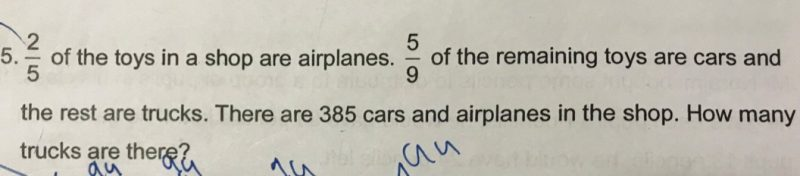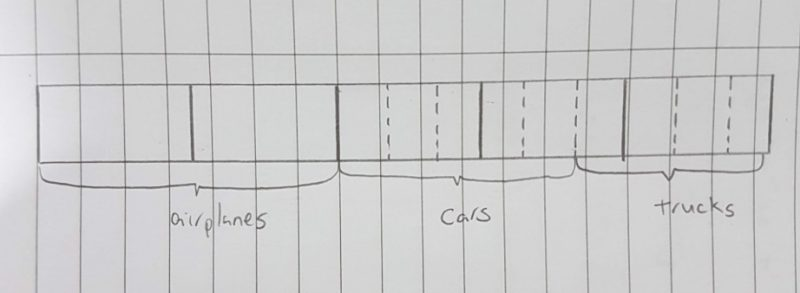# QuestionHi,

Kindly help on this question.

Thank youOnce you draw it out, it will be quite clear.

Total units for Airplanes and Cars = 6 + 5                                                                                                                                                                             = 11

11 units = 385

1 unit = 35

4 units = 140

**Let me know if you need more explanation on this question.

0 Replies 1 Like ✔Accepted Answer

A : Left : Total
2  : 3       : 5
6  : 9       : 15

C : T : Left
5  : 4  : 9

A + C = 6u + 5u
= 11u
11u = 385
1u = 35
4u = 140 (Ans)

0 Replies 1 Like